# Important Questions on Motion in a plane for JEE Main & JEE Advanced

(A) A plank of length L is moving in the direction of x-axis in the X-Y plane. A point A is located on the left hand side of the plank. Position of point A at t=0 is (L,0). The plank is moving in the right direction with velocity v
Question 1
A person standing at point A at t=0 throws a stone with velocity v and at an angle 600 with X axis. Find the co-ordinates of the maximum height reach by the stone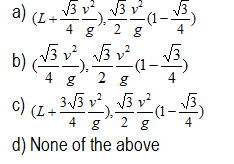Question 2
Find the velocity vector at the maximum height if i and j  are the unit vectors along X and Y axis respectively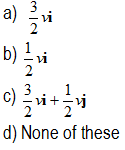Question 3
Find the co-ordinates of the stone where its hit the X-axis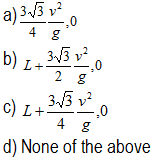Question 4
Find the velocity vector of the stone when it hits X-axis.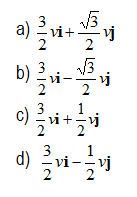Question 5
Find the co-ordinates of the point A on the plank when the stone hit the X-axis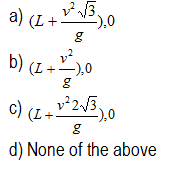Question 6
Find the maximum value of velocity v so as to land the stone on the plank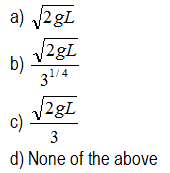(B) Two ring X and Y are put on two vertical stationary rods AB and AB. An inextensible thread is fixed at point A and on ring X and is passed through ring Y.
The system is shown below in Figure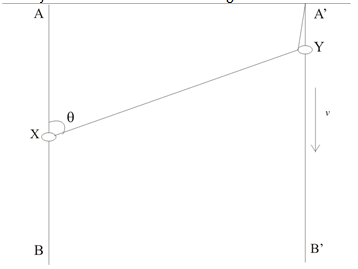Question 7
The ring X moves with constant velocity v downwards. The angle AXY is θ. Find the velocity of the ring Y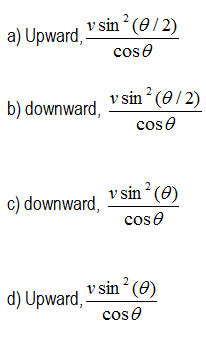Question 8
Find the velocity of ring Y with respect to ring X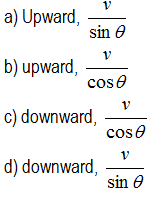(C) A man X can swim at a speed of 10 m/s with respect to river . He wants to cross the river which is 50 m wide and has a current of 5 m/s
If he wishes to land on the other bank at a point directly across the river from his starting point
Question 9
Velocity of man with respect to the person standing on the bank
a) 5
b) 10
c) 6
d)5√3

Question 10
Find the angle made by the man from the current
a) 1200
b) 600
c) 300
d) 900
Question 11
Find the time taken by the man to cross the river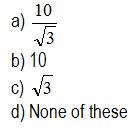(D)Now if he instead decides to cross the river in shortest possible time
Question 12
what direction it should swim
a) 900 to current
b) 300 to current
c) 600 to current
d) none of these
Question 13
How much time
a) 5
b) 10
c) 6
d) 4
Question 14
How much distance it will land from his starting point
a) 30
b) 20
c) 25
d) none of these
(E)The radius vector describing the position of the particle A relative to origin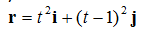Question 15
Find the rectangular components of the average velocity in the time interval between t and t+?t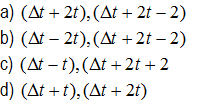Question 16
Which of the following statements are true about the motion
a) The particle is experiencing uniform acceleration motion
b) The particle starts at y axis and touched x axis in 1sec
c) The initial velocity is towards negative Y axis
d) The velocity at t=1 is towards positive x axis
(F) A particle is moving along a parabola given by
y=x2
So that at any time vx=3 m/s
Question 17
Find the magnitude and direction of velocity at x=1/3 m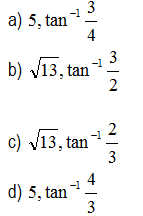Question 18
Find the acceleration at x=1/3 (both magnitude and direction)
a) 18 m/sec2 ,900
b) 14 m/sec2 ,600
c) 18 m/sec2 ,600
d) 14 m/sec2 ,900
(G)An object is fired up on the inclined place of angle α . The object is fired with velocity v0 and angle of elevation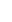θ with the horizontal
Question 19
Find the range on the inclined plane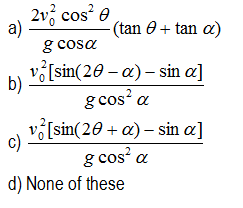Question 20
Find the relation between  andα for which object will have maximum range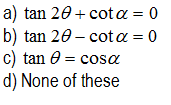Answers
1. (c)
2. (a)
3. (b)
4. (b)
5. (a)
6. (b)
7. (a)
8. (b)
9. (d)
10. (a)
11. (a)
12. (a)
13. (a)
14. (c)
15. (a)
16. (a),(b),(c),(d)
17. (c)
18. (a)
19. (b)
20. (a)

Solutions

link to this page by copying the following text